# RD Sharma Solutions for Class 9 Maths Chapter 3 Rationalisation

## RD Sharma Solutions for Class 9 Maths Chapter 3 – Free PDF Download

RD Sharma Solutions for Class 9 Maths Chapter 3 – Rationalisation is one of the most important chapters in Class 9. Students learn about different algebraic identities and rationalisation of the denominator in  RD Sharma Solutions for Class 9 Chapter 3. A rationalisation is a process by which radicals in the denominator of a fraction are eliminated. In this chapter, students will learn to simplify algebraic expressions using identities. Students are advised to memorize all the identities before they attempt any rationalisation question. To download the PDF of Chapter 3 click on the below link.

RD Sharma Solutions are useful for students as it helps them in scoring high marks in the examination. These solutions are prepared by subject matter experts at BYJU’S, describing the complete method of solving problems. In order to excel in exams, students can view and download the PDF of RD Sharma Solutions for Class 9 Maths Chapter 3.

## Download PDF of RD Sharma Solutions for Class 9 Maths Chapter 3 Rationalisation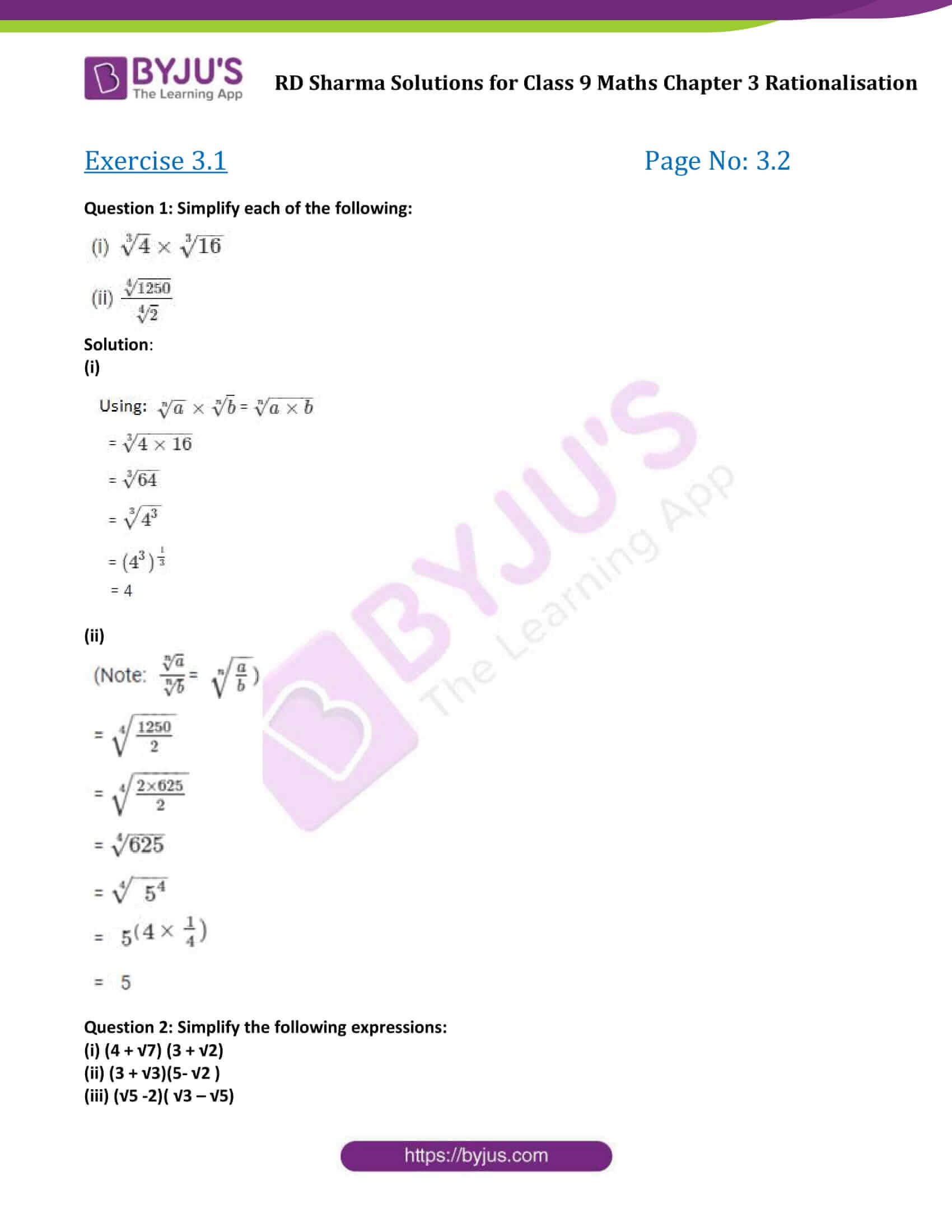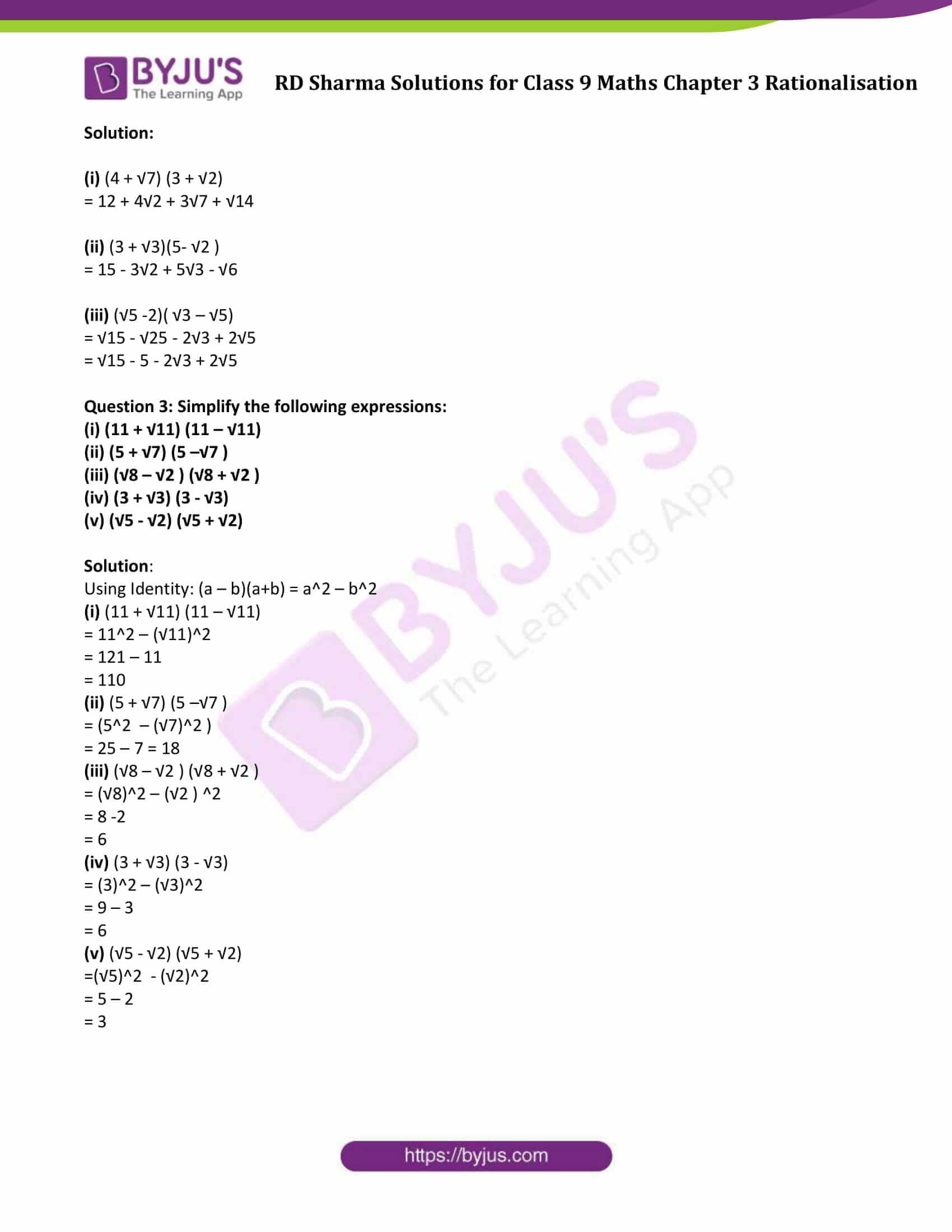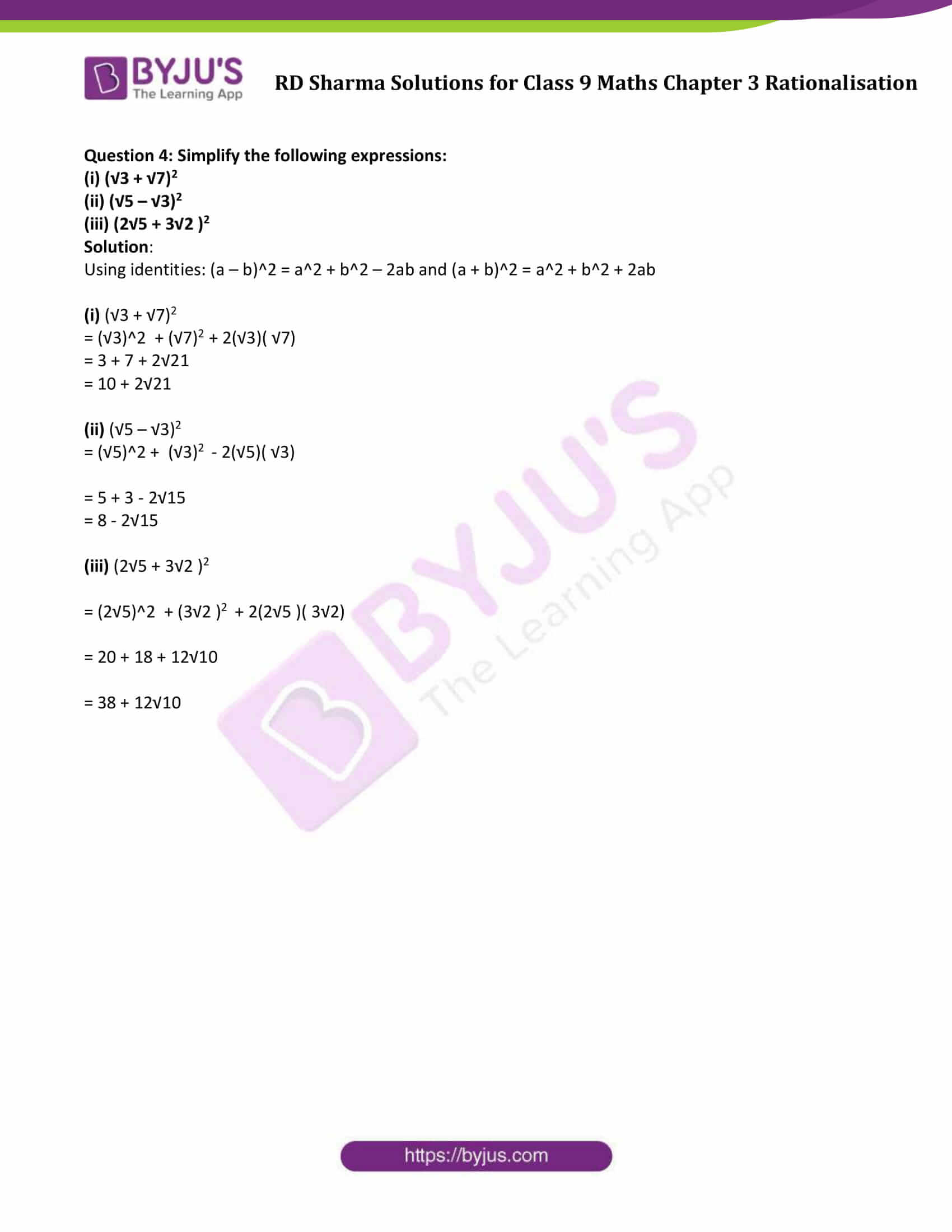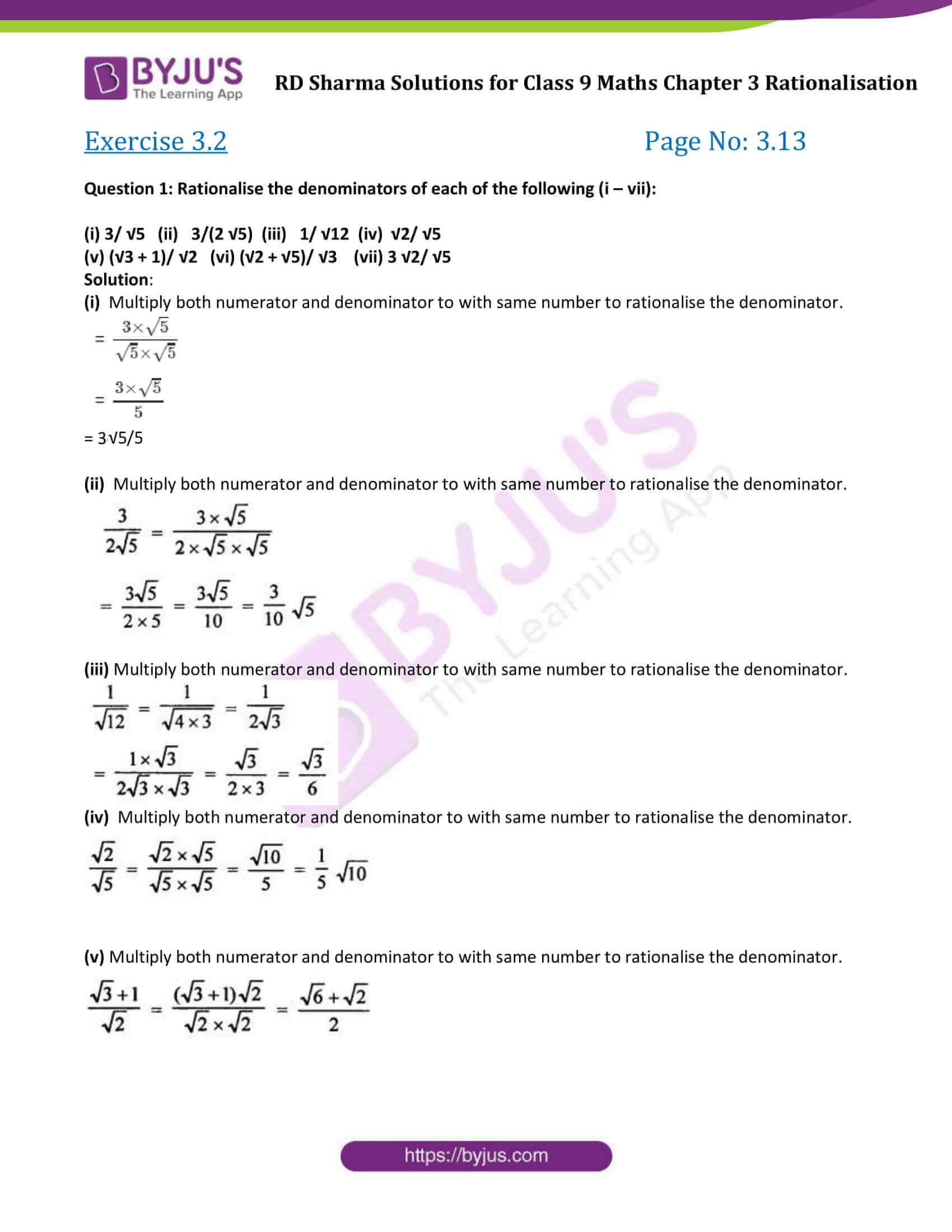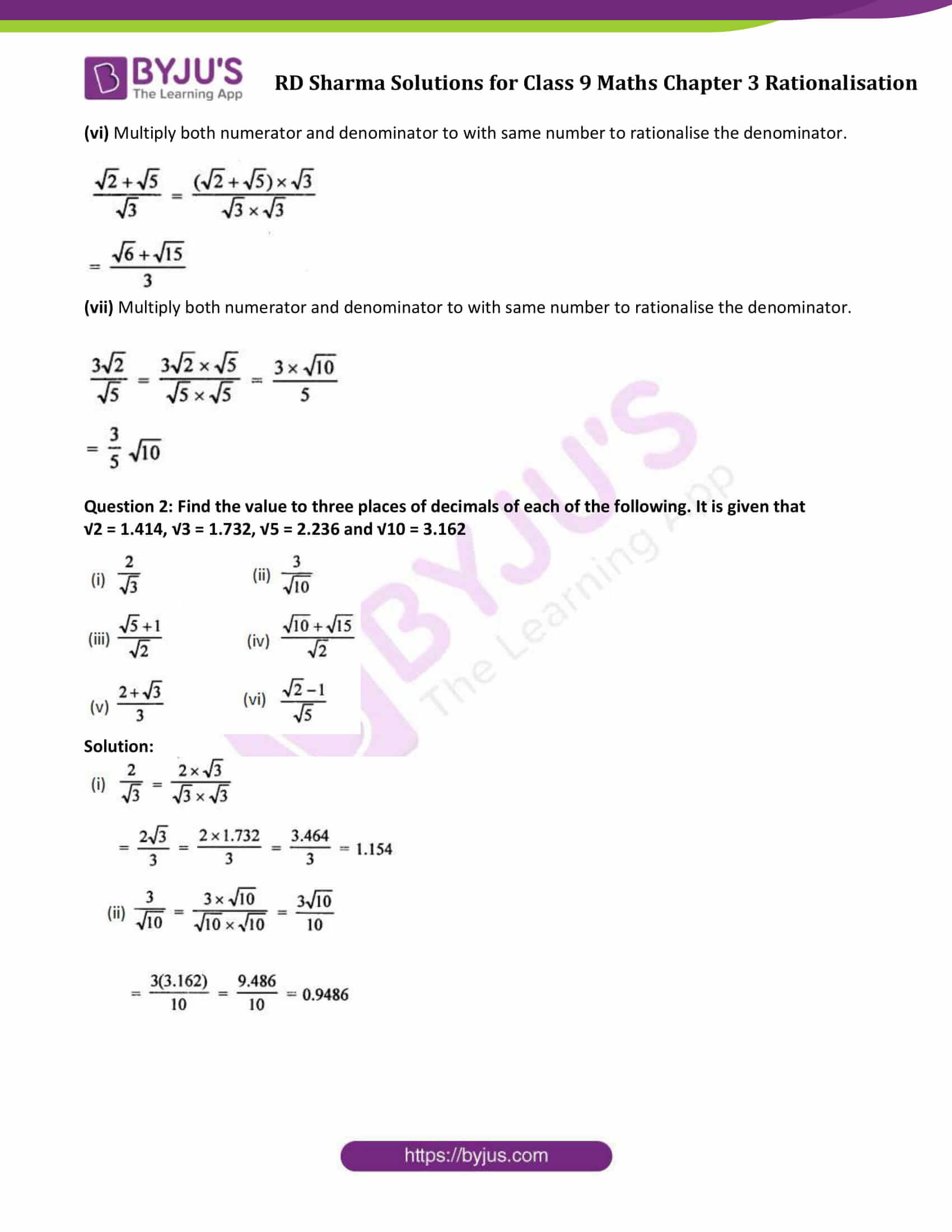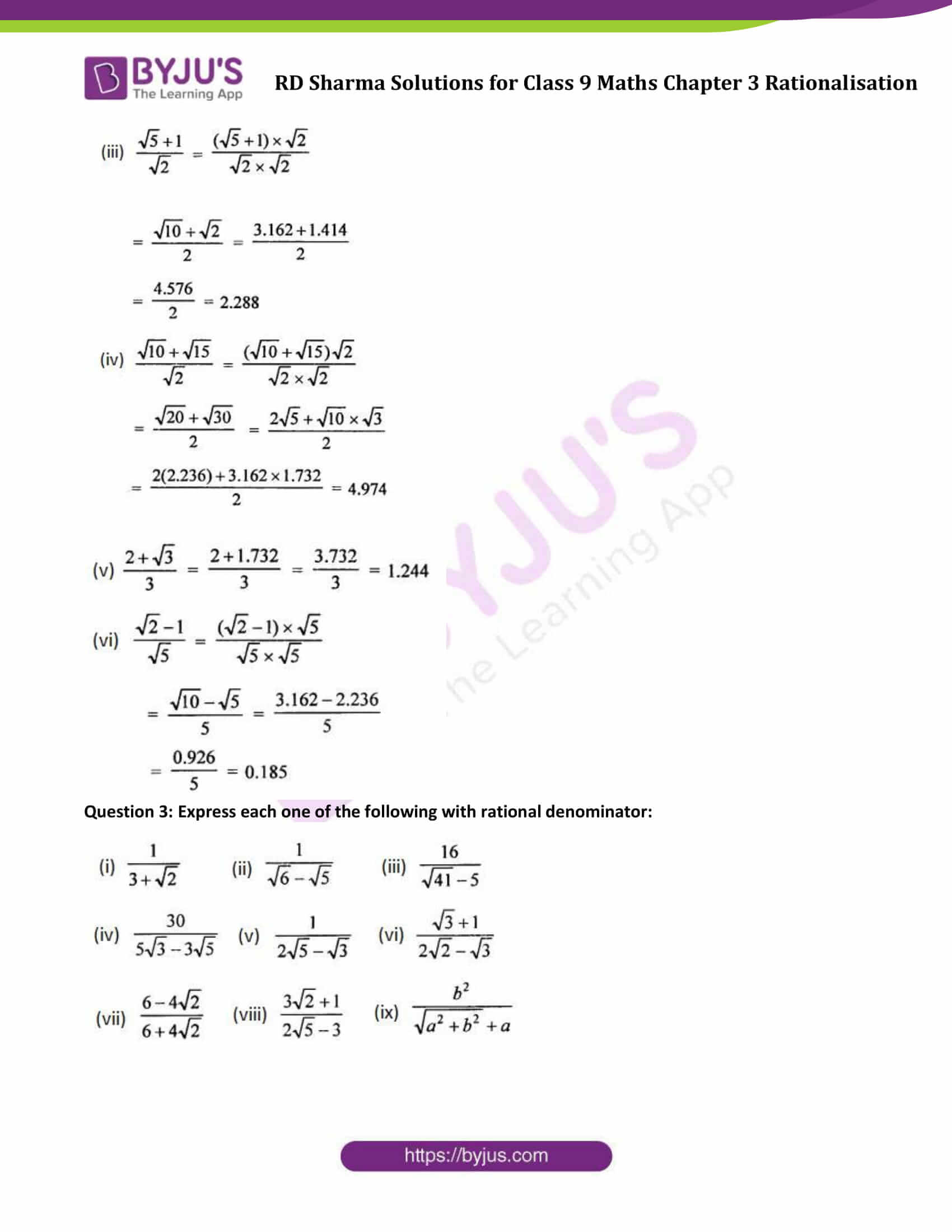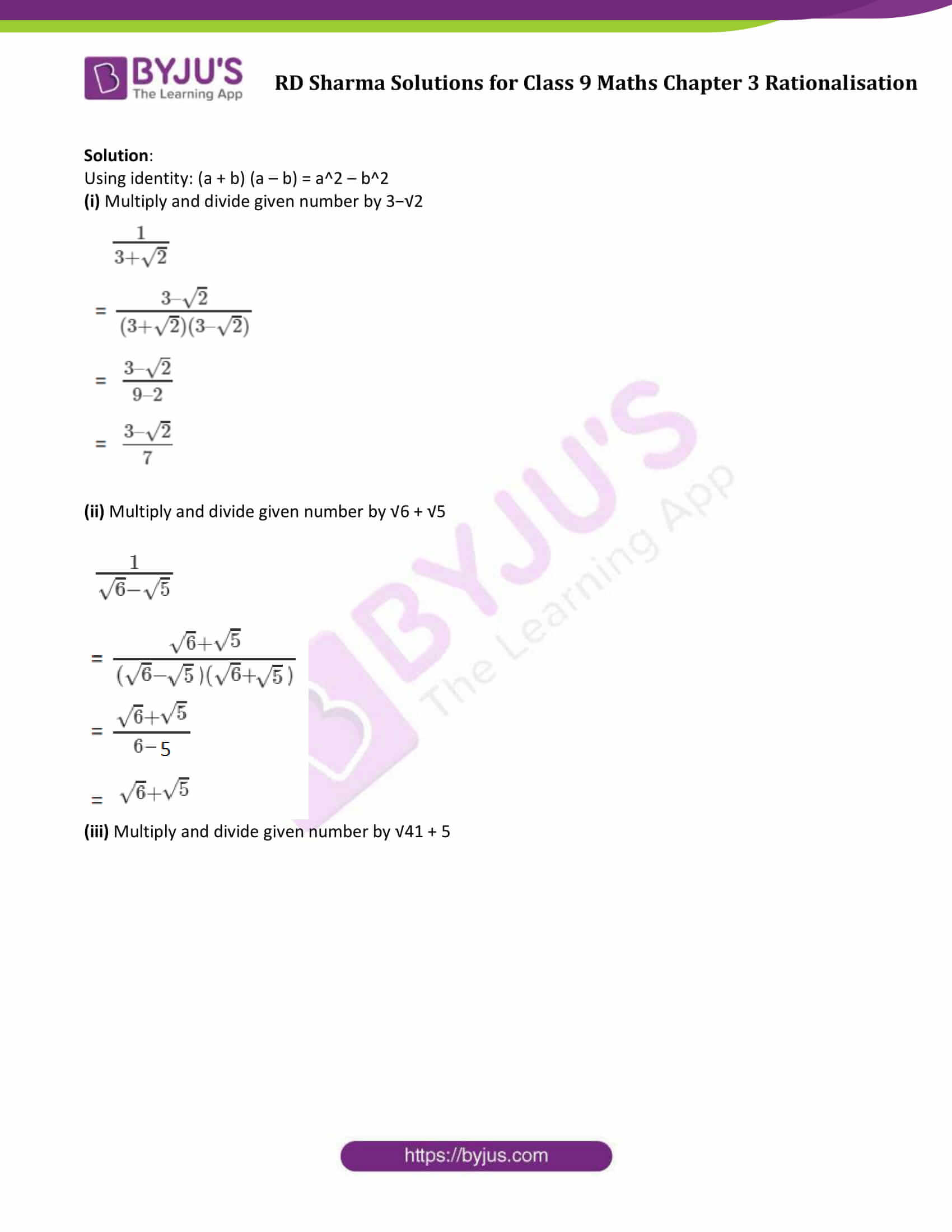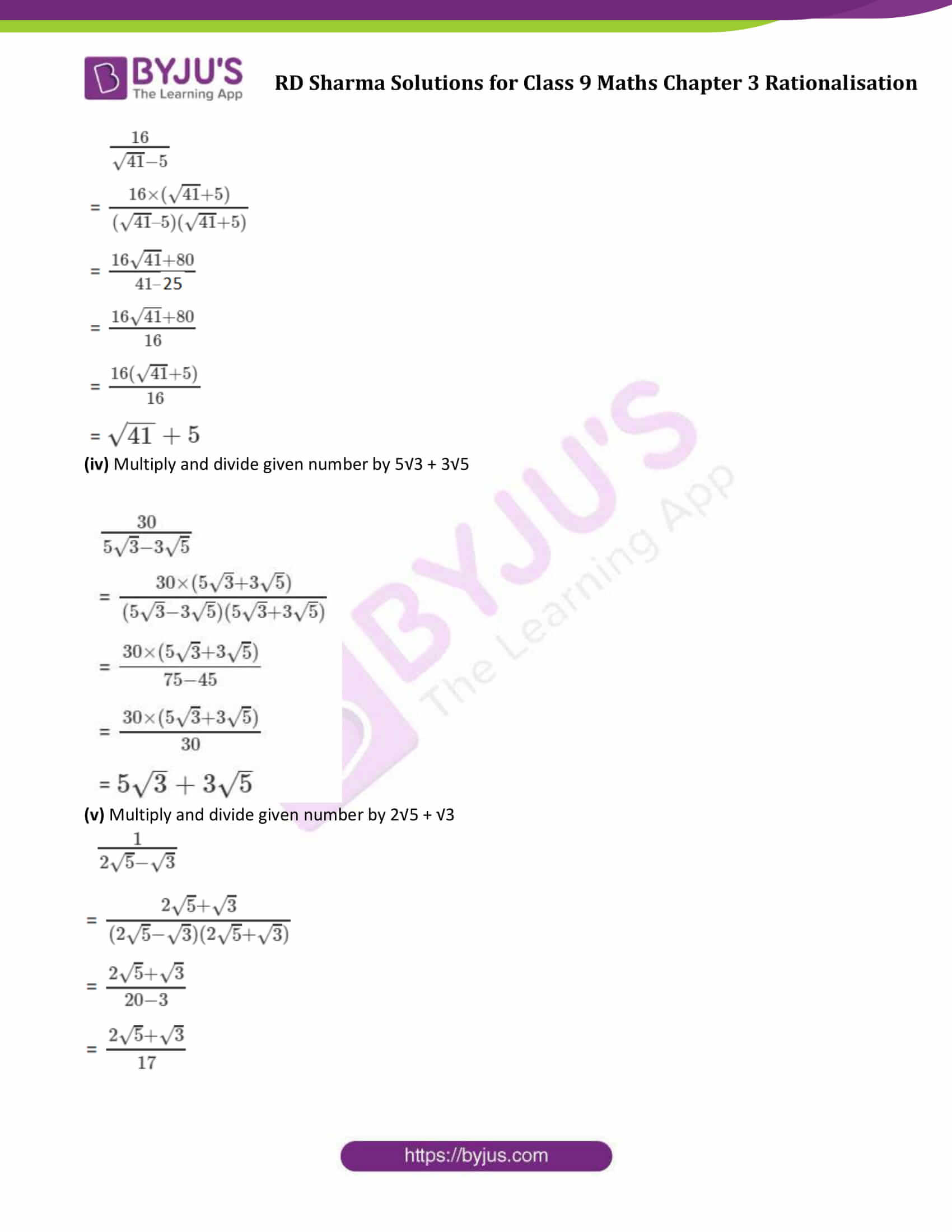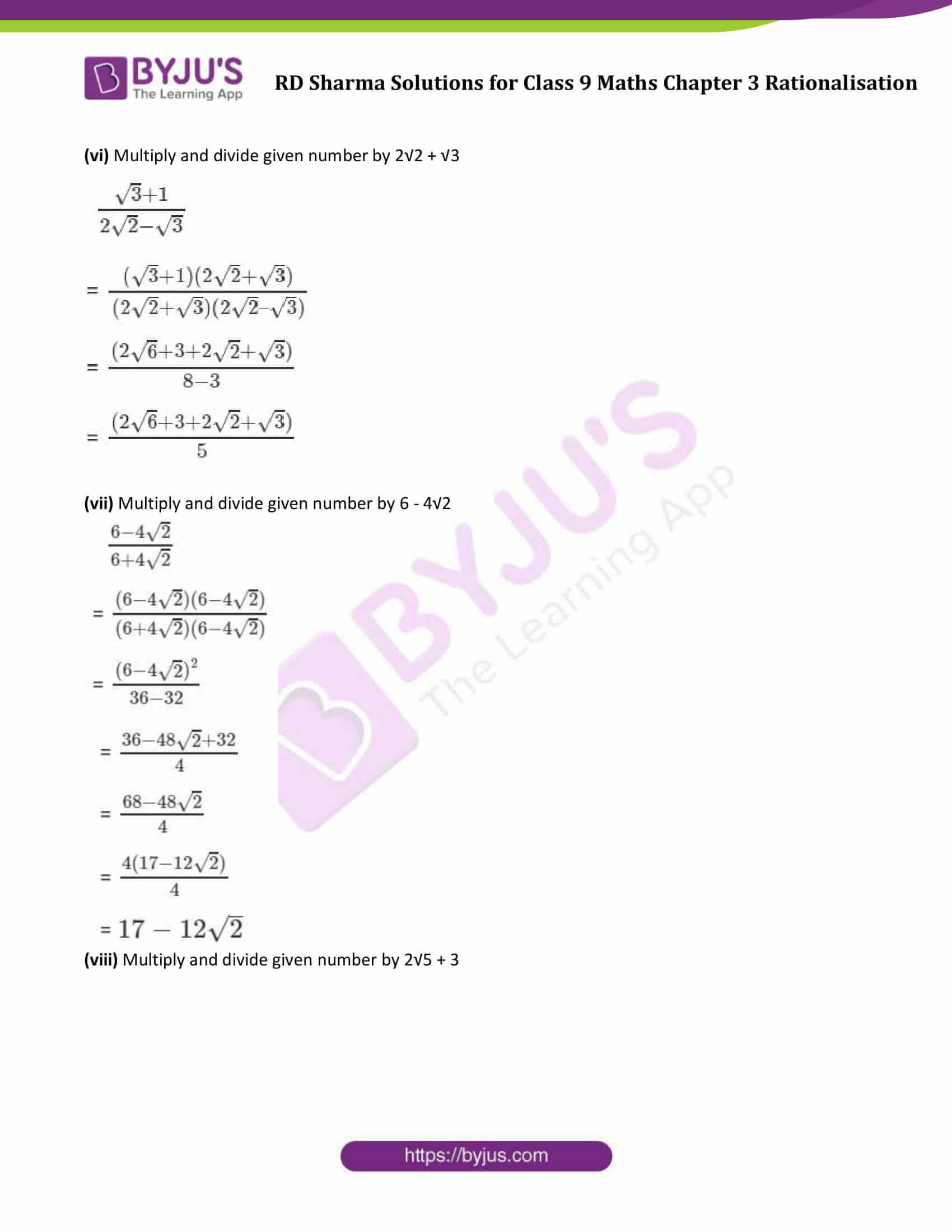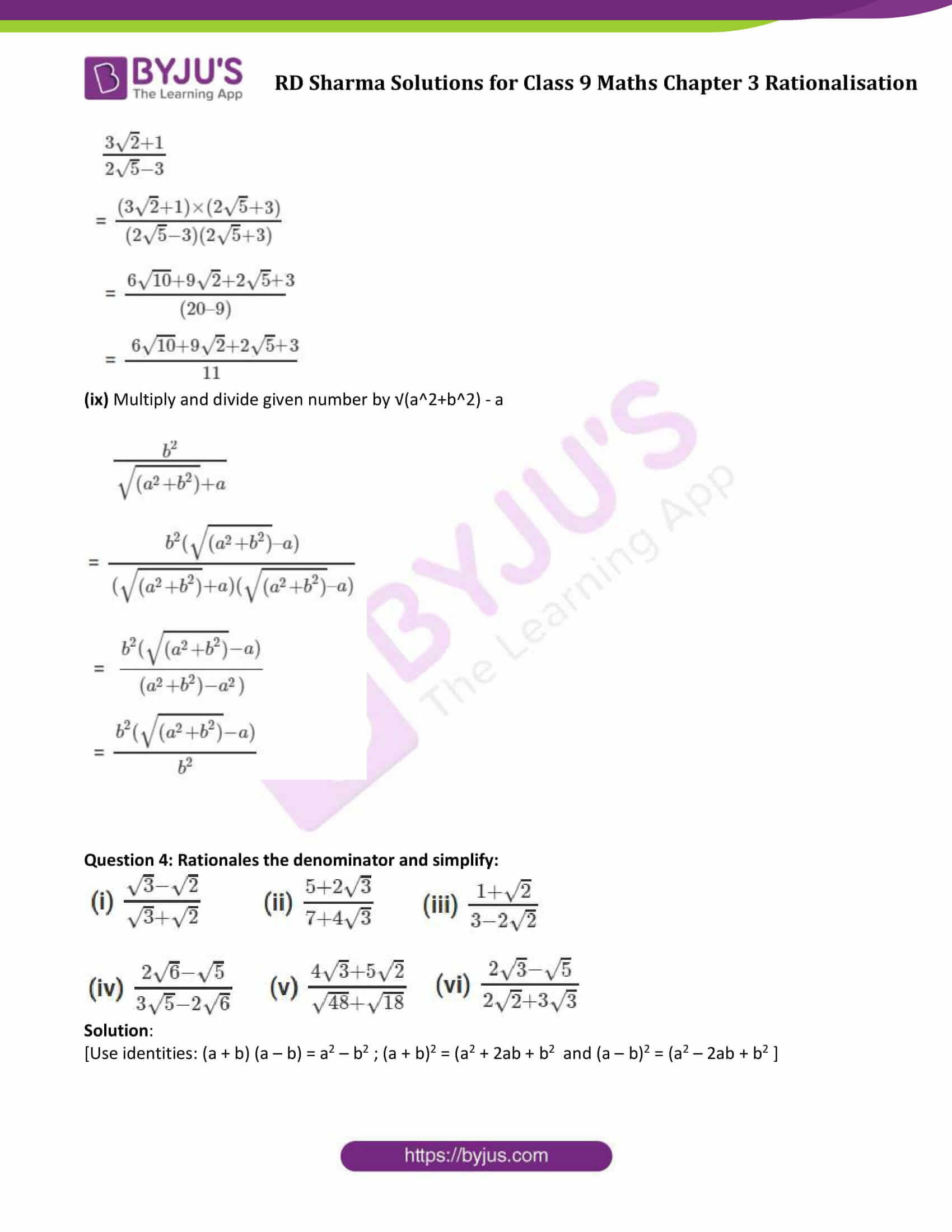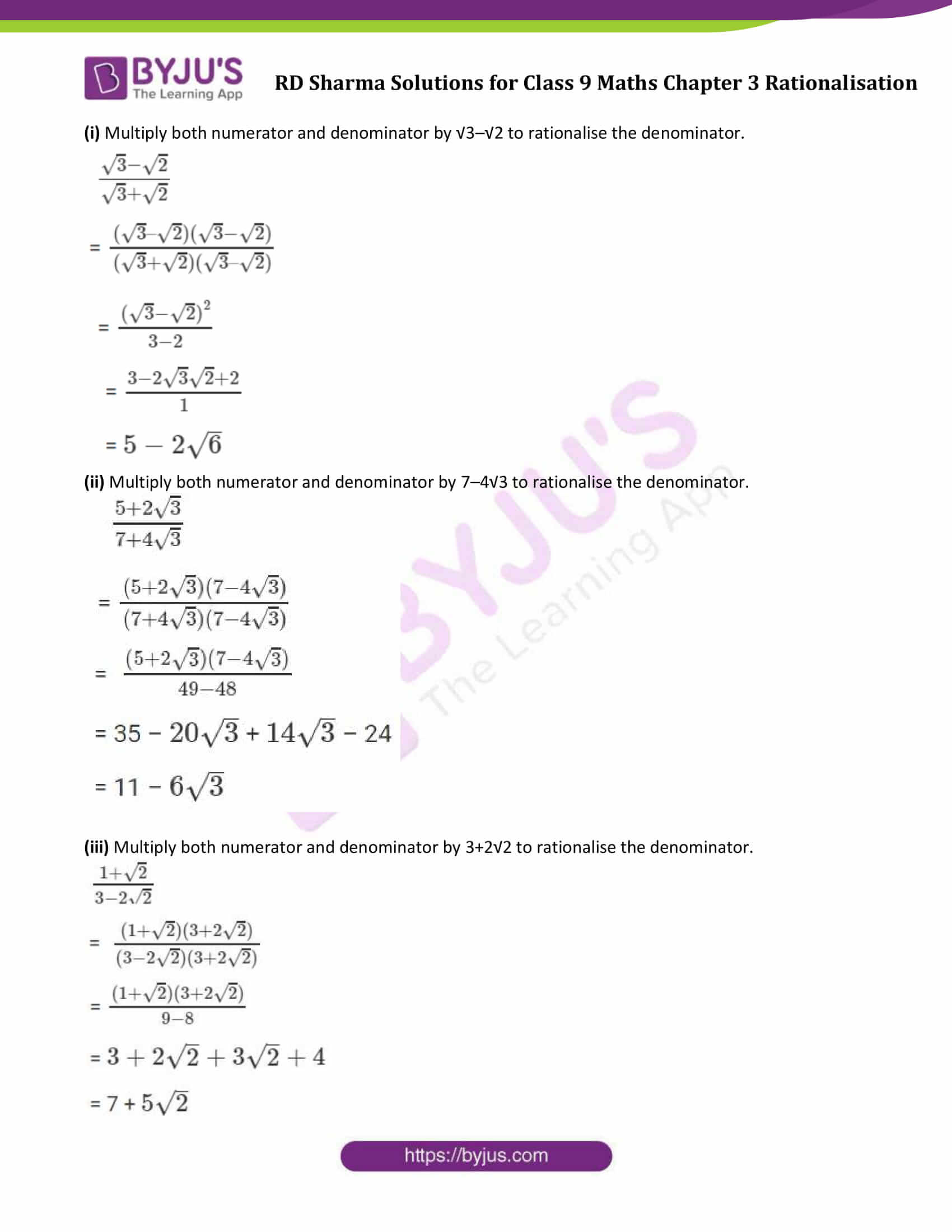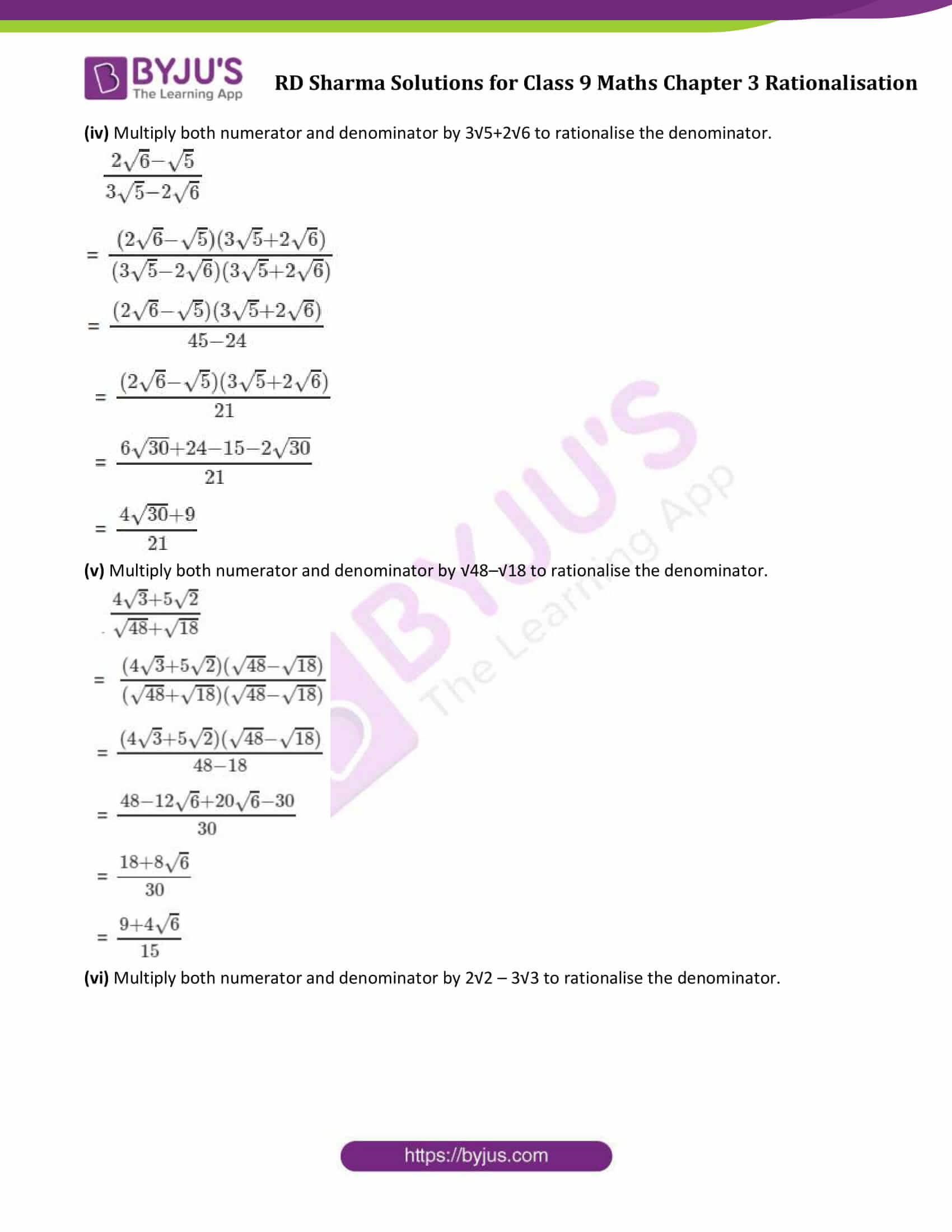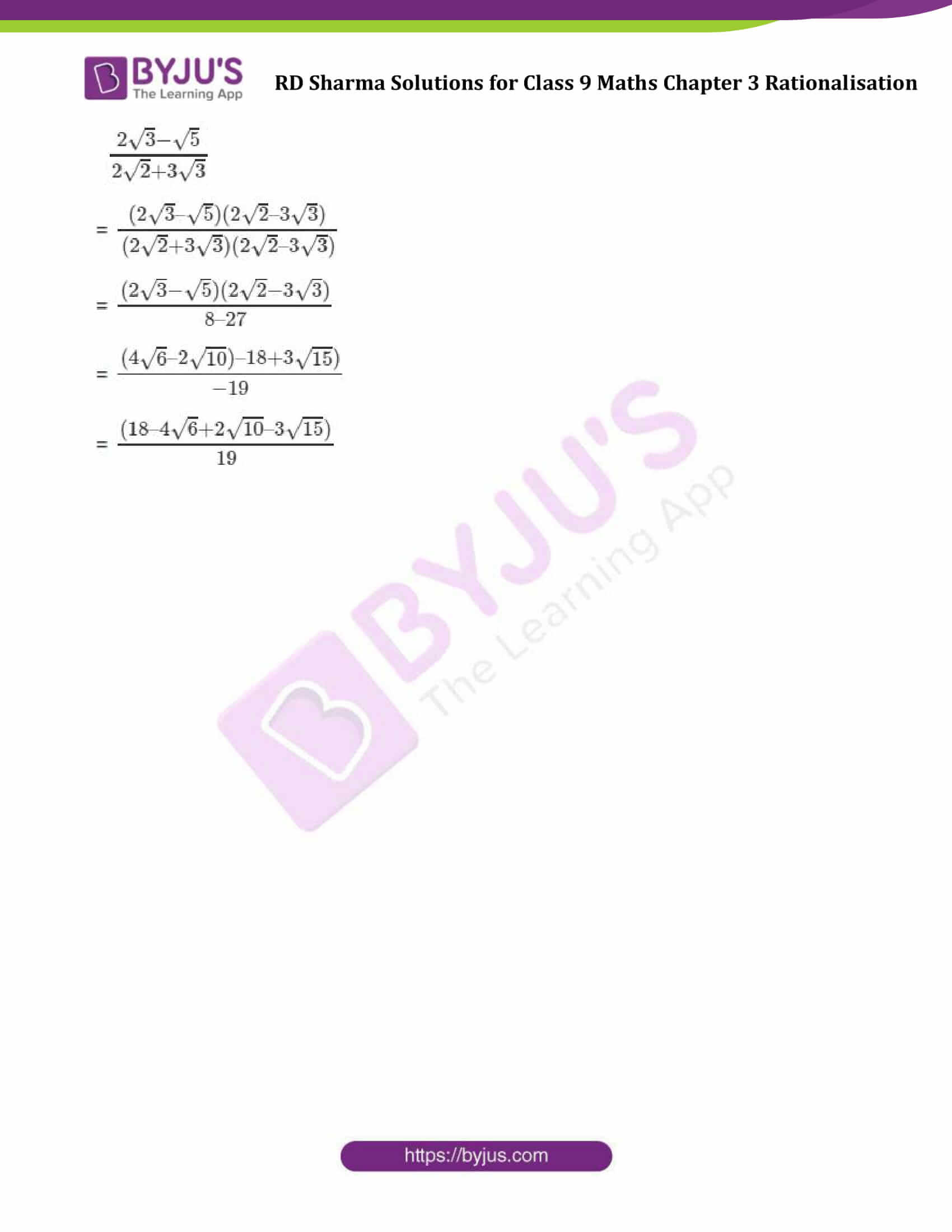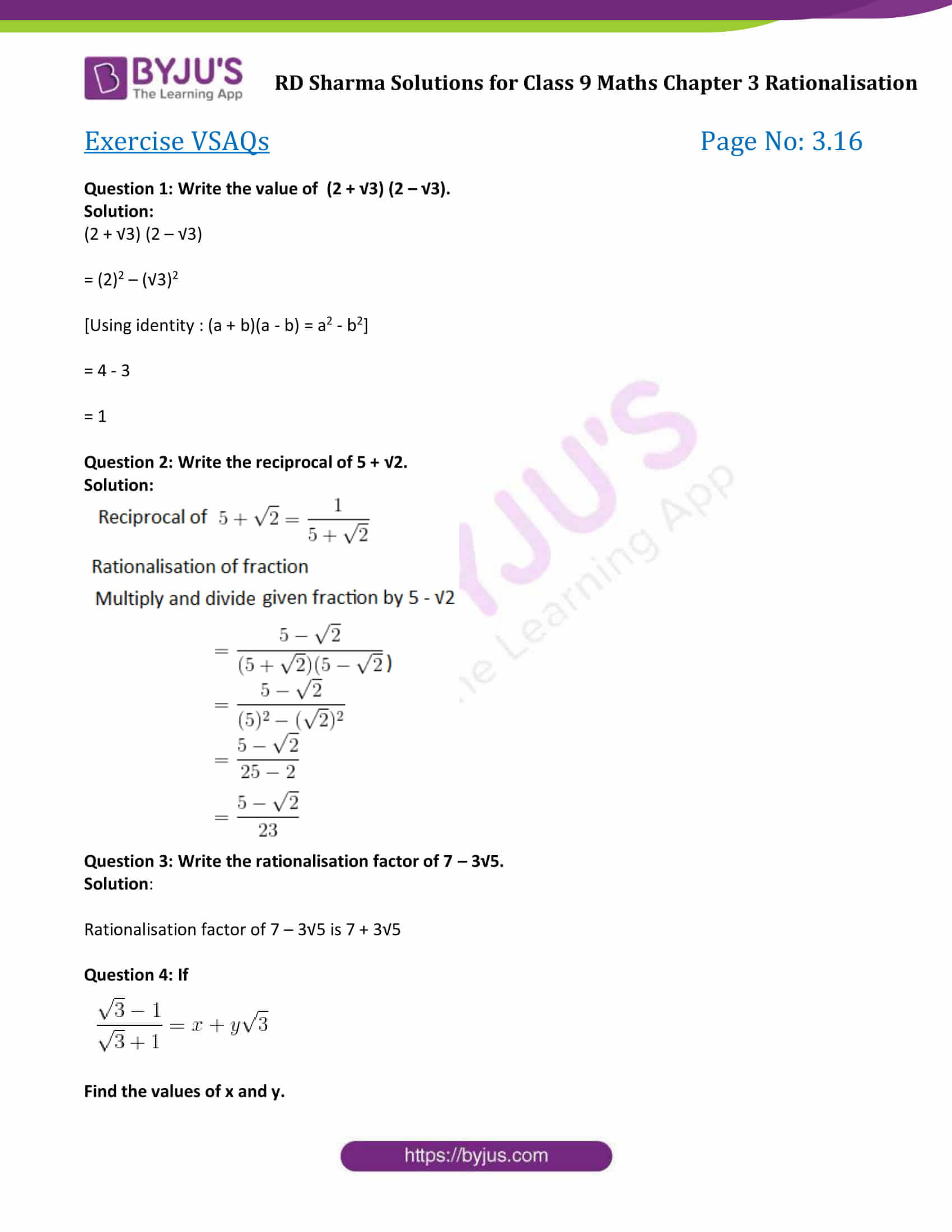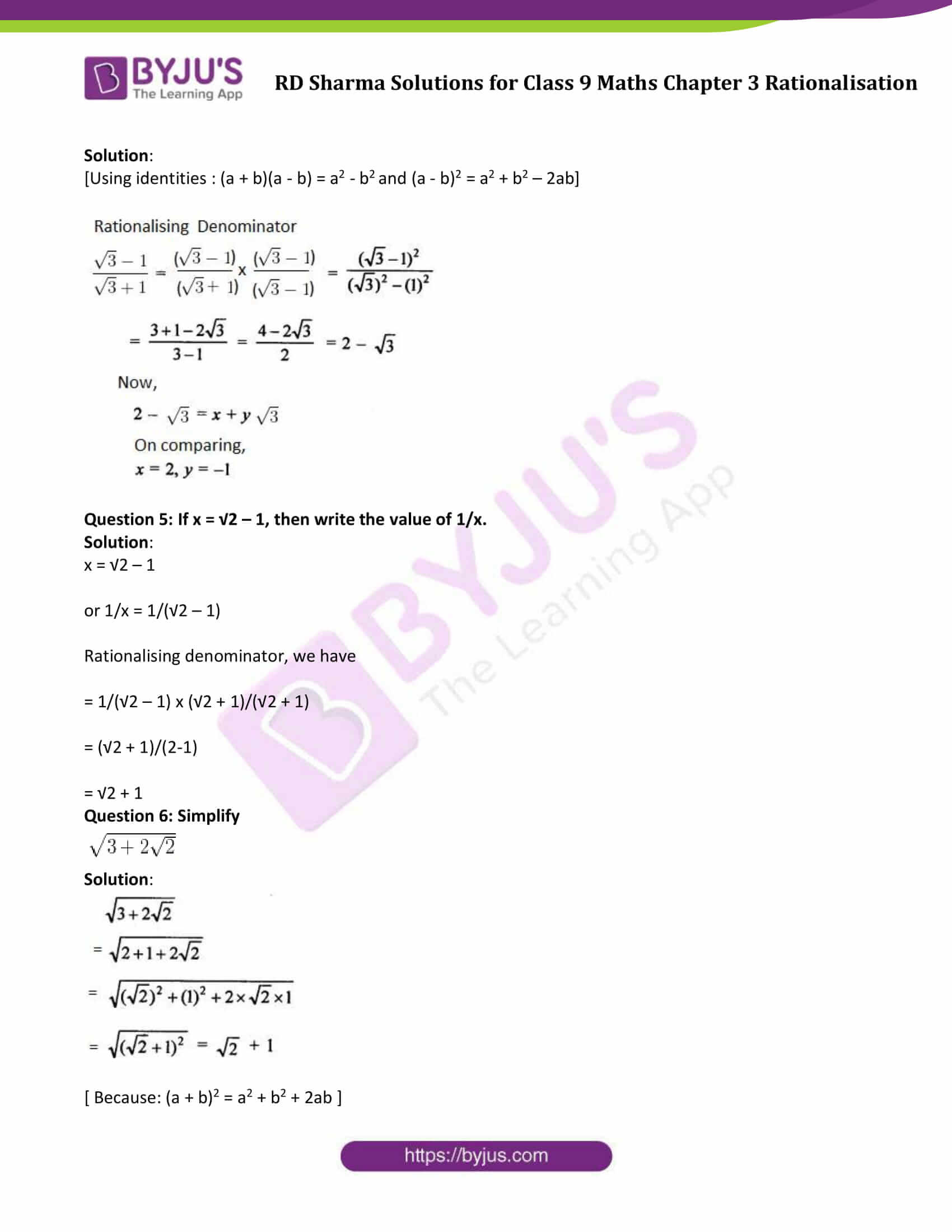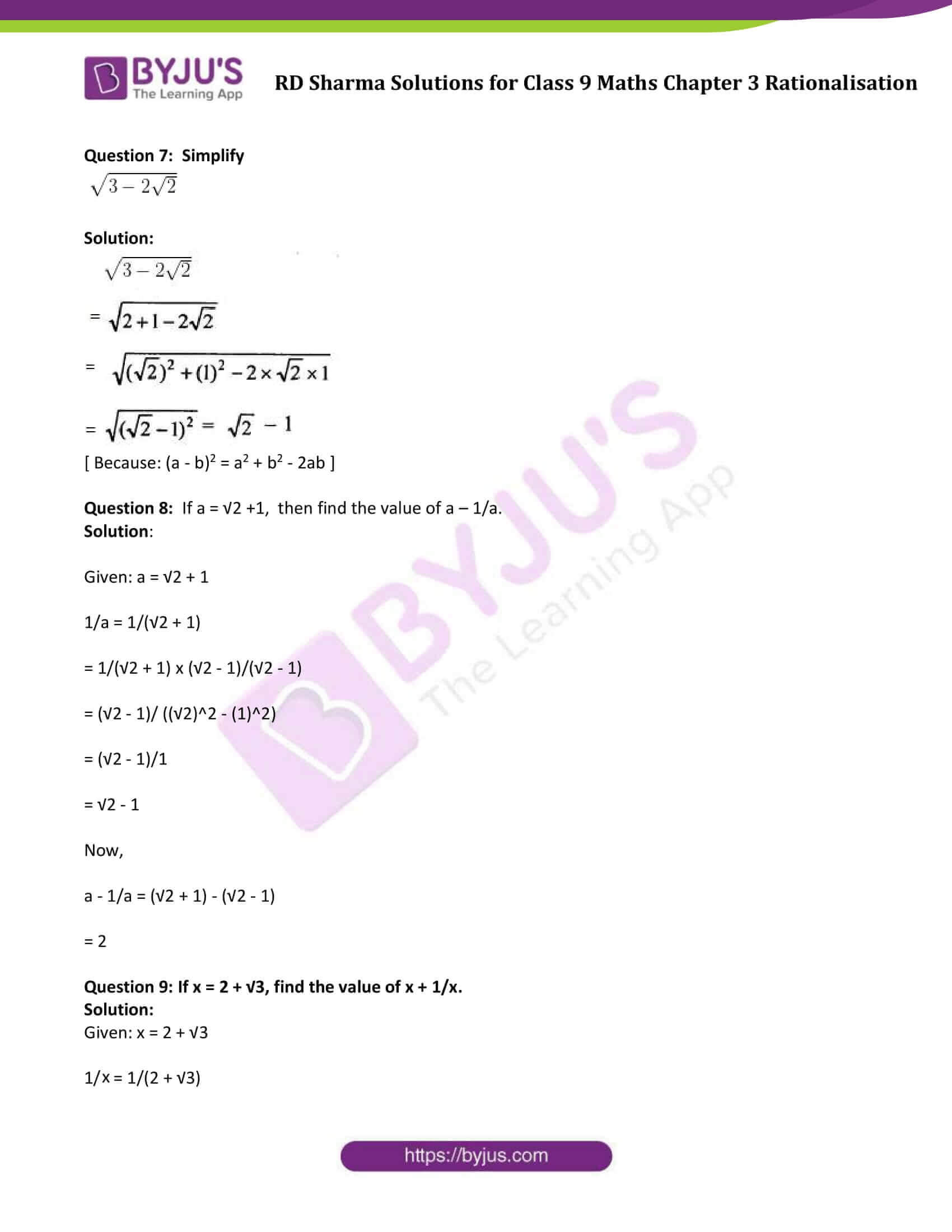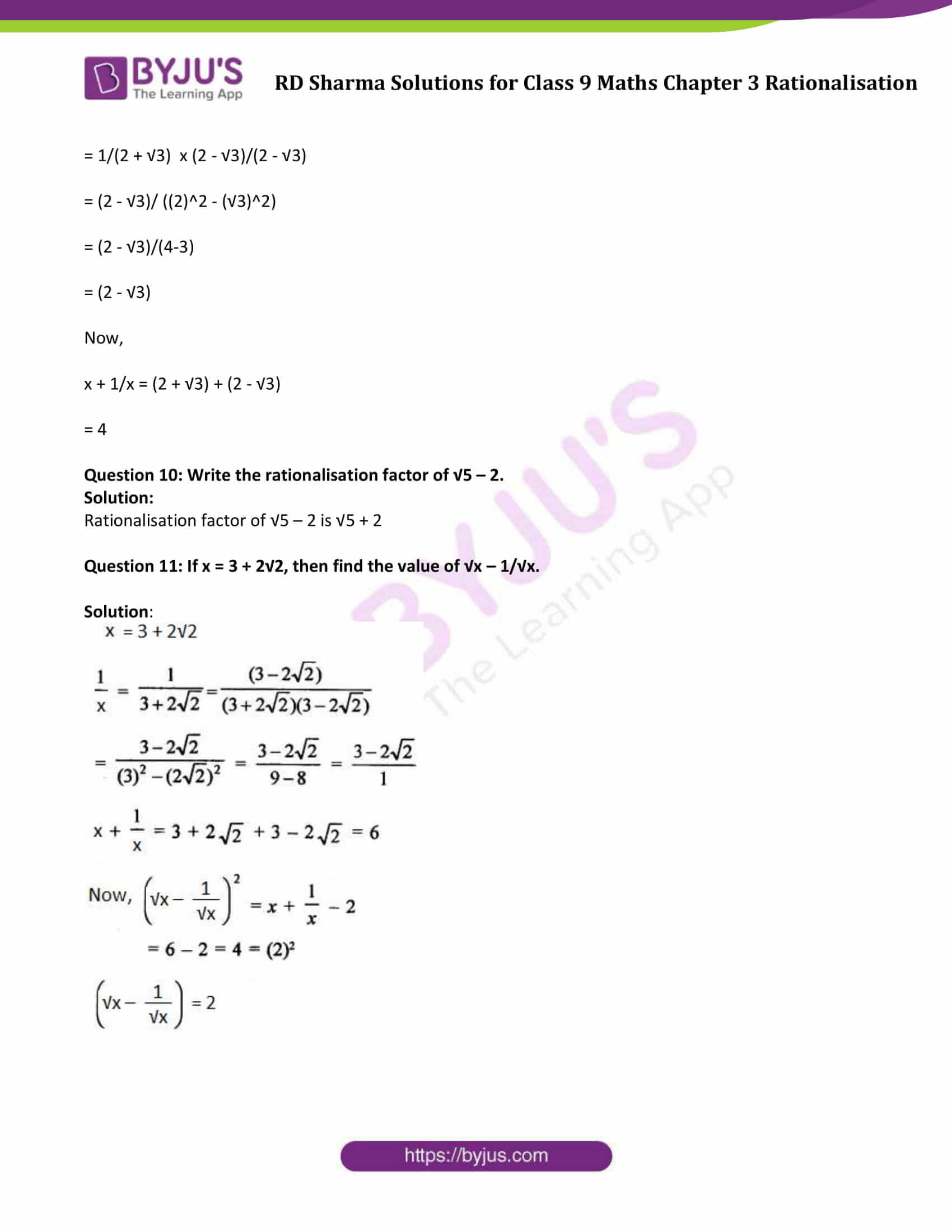### Exercise 3.1

Question 1: Simplify each of the following:Solution:

(i)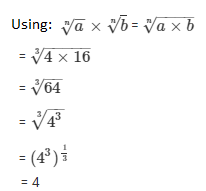(ii)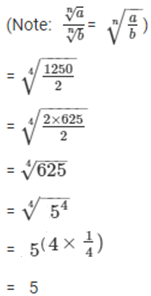Question 2: Simplify the following expressions:

(i) (4 + √7) (3 + √2)

(ii) (3 + √3)(5- √2 )

(iii) (√5 -2)( √3 – √5)

Solution:

(i) (4 + √7) (3 + √2)

= 12 + 4√2 + 3√7 + √14

(ii) (3 + √3)(5- √2 )

= 15 – 3√2 + 5√3 – √6

(iii) (√5 -2)( √3 – √5)

= √15 – √25 – 2√3 + 2√5

= √15 – 5 – 2√3 + 2√5

Question 3: Simplify the following expressions:

(i) (11 + √11) (11 – √11)

(ii) (5 + √7) (5 –√7 )

(iii) (√8 – √2 ) (√8 + √2 )

(iv) (3 + √3) (3 – √3)

(v) (√5 – √2) (√5 + √2)

Solution:

Using Identity: (a – b)(a+b) = a2 – b2

(i) (11 + √11) (11 – √11)

= 112 – (√11)2

= 121 – 11

= 110

(ii) (5 + √7) (5 –√7 )

= (52 – (√7)2 )

= 25 – 7 = 18

(iii) (√8 – √2 ) (√8 + √2 )

= (√8)2 – (√2 ) 2

= 8 -2

= 6

(iv) (3 + √3) (3 – √3)

= (3)2 – (√3)2

= 9 – 3

= 6

(v) (√5 – √2) (√5 + √2)

=(√5)2 – (√2)2

= 5 – 2

= 3

Question 4: Simplify the following expressions:

(i) (√3 + √7)2

(ii) (√5 – √3)2

(iii) (2√5 + 3√2 )2

Solution:

Using identities: (a – b)2 = a2 + b2 – 2ab and (a + b)2 = a2 + b2 + 2ab

(i) (√3 + √7)2

= (√3)2 + (√7)2 + 2(√3)( √7)

= 3 + 7 + 2√21

= 10 + 2√21

(ii) (√5 – √3)2

= (√5)2 + (√3)2 – 2(√5)( √3)

= 5 + 3 – 2√15

= 8 – 2√15

(iii) (2√5 + 3√2 )2

= (2√5)2 + (3√2 )2 + 2(2√5 )( 3√2)

= 20 + 18 + 12√10

= 38 + 12√10

### Exercise 3.2

Question 1: Rationalise the denominators of each of the following (i – vii):

(i) 3/ √5 (ii) 3/(2 √5) (iii) 1/ √12 (iv) √2/ √5

(v) (√3 + 1)/ √2 (vi) (√2 + √5)/ √3 (vii) 3 √2/ √5

Solution:

(i) Multiply both numerator and denominator to with same number to rationalise the denominator.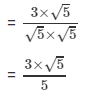= 3√5/5

(ii) Multiply both numerator and denominator to with same number to rationalise the denominator.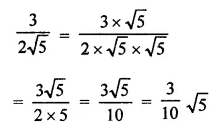(iii) Multiply both numerator and denominator to with same number to rationalise the denominator.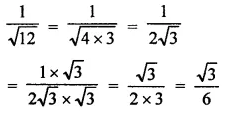(iv) Multiply both numerator and denominator to with same number to rationalise the denominator.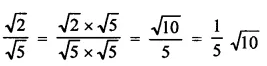(v) Multiply both numerator and denominator to with same number to rationalise the denominator.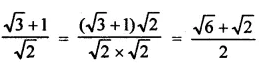(vi) Multiply both numerator and denominator to with same number to rationalise the denominator.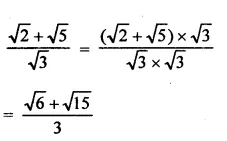(vii) Multiply both numerator and denominator to with same number to rationalise the denominator.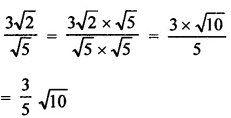Question 2: Find the value to three places of decimals of each of the following. It is given that

√2 = 1.414, √3 = 1.732, √5 = 2.236 and √10 = 3.162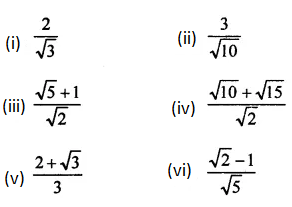Solution: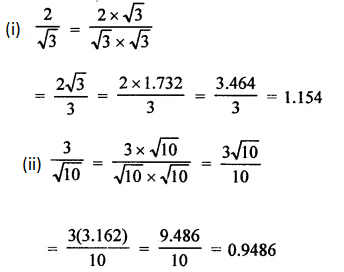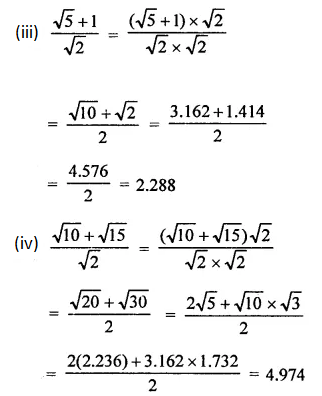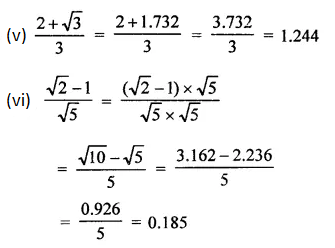Question 3: Express each one of the following with rational denominator: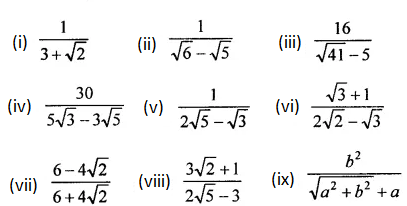Solution:

Using identity: (a + b) (a – b) = a2 – b2

(i) Multiply and divide given number by 3−√2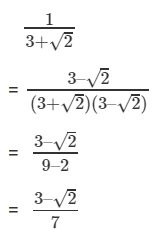(ii) Multiply and divide given number by √6 + √5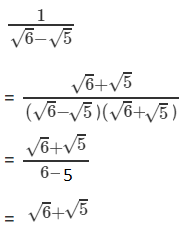(iii) Multiply and divide given number by √41 + 5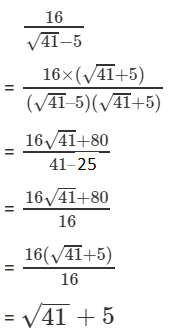(iv) Multiply and divide given number by 5√3 + 3√5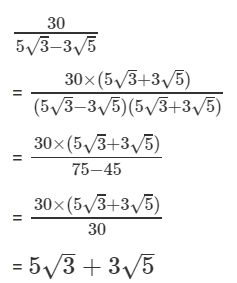(v) Multiply and divide given number by 2√5 + √3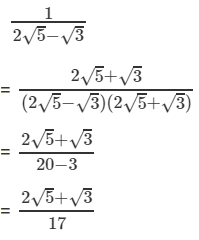(vi) Multiply and divide given number by 2√2 + √3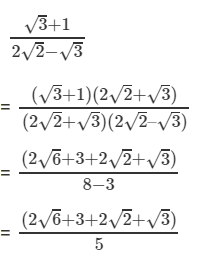(vii) Multiply and divide given number by 6 – 4√2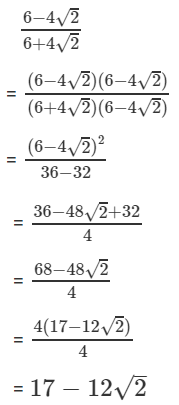(viii) Multiply and divide given number by 2√5 + 3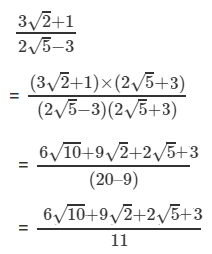(ix) Multiply and divide given number by √(a2+b2) – a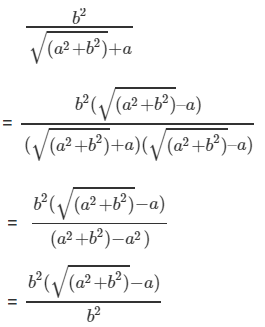Question 4: Rationales the denominator and simplify: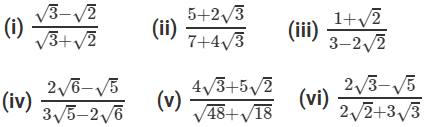Solution:

[Use identities: (a + b) (a – b) = a2 – b2 ; (a + b)2 = (a2 + 2ab + b2 and (a – b)2 = (a2 – 2ab + b2 ]

(i) Multiply both numerator and denominator by √3–√2 to rationalise the denominator.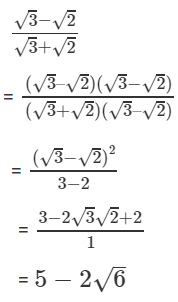(ii) Multiply both numerator and denominator by 7–4√3 to rationalise the denominator.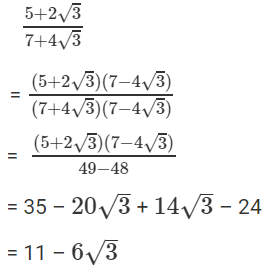(iii) Multiply both numerator and denominator by 3+2√2 to rationalise the denominator.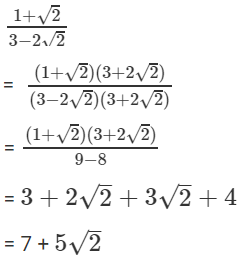(iv) Multiply both numerator and denominator by 3√5+2√6 to rationalise the denominator.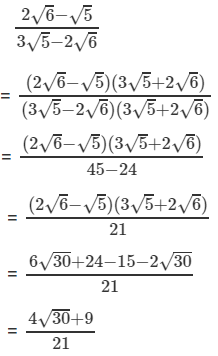(v) Multiply both numerator and denominator by √48–√18 to rationalise the denominator.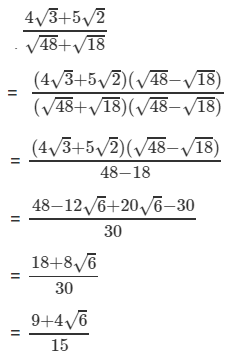(vi) Multiply both numerator and denominator by 2√2 – 3√3 to rationalise the denominator.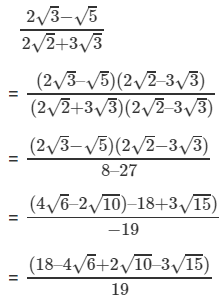### Exercise VSAQs

Question 1: Write the value of (2 + √3) (2 – √3).

Solution:

(2 + √3) (2 – √3)

= (2)2 – (√3)2

[Using identity : (a + b)(a – b) = a2 – b2]

= 4 – 3

= 1

Question 2: Write the reciprocal of 5 + √2.

Solution: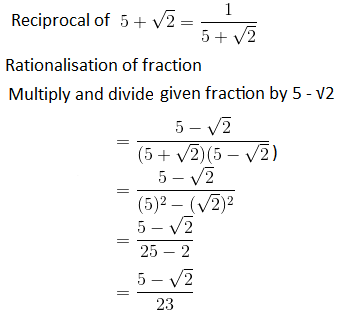Question 3: Write the rationalisation factor of 7 – 3√5.

Solution:

Rationalisation factor of 7 – 3√5 is 7 + 3√5

Question 4: If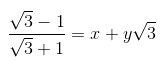Find the values of x and y.

Solution:

[Using identities : (a + b)(a – b) = a2 – b2 and (a – b)2 = a2 + b2 – 2ab]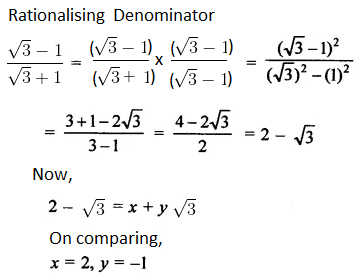Question 5: If x = √2 – 1, then write the value of 1/x.

Solution:

x = √2 – 1

or 1/x = 1/(√2 – 1)

Rationalising denominator, we have

= 1/(√2 – 1) x (√2 + 1)/(√2 + 1)

= (√2 + 1)/(2-1)

= √2 + 1

Question 6: Simplify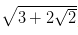Solution: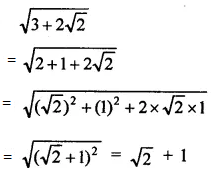[ Because: (a + b)2 = a2 + b2 + 2ab ]

Question 7: Simplify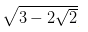Solution: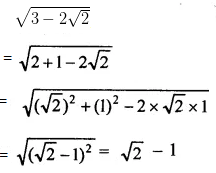[ Because: (a – b)2 = a2 + b2 – 2ab ]

Question 8: If a = √2 +1, then find the value of a – 1/a.

Solution:

Given: a = √2 + 1

1/a = 1/(√2 + 1)

= 1/(√2 + 1) x (√2 – 1)/(√2 – 1)

= (√2 – 1)/ ((√2)2 – (1)2)

= (√2 – 1)/1

= √2 – 1

Now,

a – 1/a = (√2 + 1) – (√2 – 1)

= 2

Question 9: If x = 2 + √3, find the value of x + 1/x.

Solution:

Given: x = 2 + √3

1/x = 1/(2 + √3)

= 1/(2 + √3) x (2 – √3)/(2 – √3)

= (2 – √3)/ ((2)2 – (√3)2)

= (2 – √3)/(4-3)

= (2 – √3)

Now,

x + 1/x = (2 + √3) + (2 – √3)

= 4

Question 10: Write the rationalisation factor of √5 – 2.

Solution:

Rationalisation factor of √5 – 2 is √5 + 2

Question 11: If x = 3 + 2√2, then find the value of √x – 1/√x.

Solution: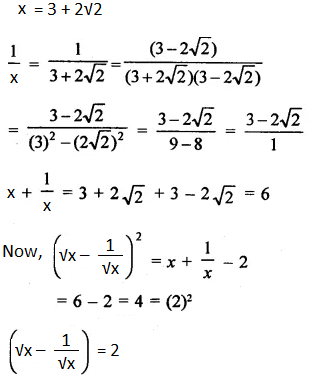### RD Sharma Solutions for Class 9 Maths Chapter 3 Rationalisation

In the 3rd chapter of Class 9 RD Sharma Solutions students will study important concepts on Rationalisation as listed below:

• Rationalisation Introduction
• Rationalisation of the denominator
• Some important algebraic identities

Students can get acquainted with various methods of solving problems with ease and face exams more confidently. Meanwhile students are suggested to solve these solutions without fail to procure great marks in their board exams.

## Frequently Asked Questions on RD Sharma Solutions for Class 9 Maths Chapter 3

### How many exercises are present in RD Sharma Solutions for Class 9 Maths Chapter 3?

RD Sharma Solutions for Class 9 Maths Chapter 3 has 2 exercises. The topics discussed in these exercises are different algebraic identities and rationalisation of the denominator. A rationalisation is a process by which radicals in the denominator of a fraction are eliminated. In this chapter, students will learn to simplify algebraic expressions using identities. Practice is an essential task to learn and score well in Mathematics. Hence the solutions are designed by BYJU’S experts to boost confidence among students in solving the complex problems effortlessly.

### Why should I opt for RD Sharma Solutions for Class 9 Maths Chapter 3?

The concepts present in RD Sharma Solutions for Class 9 Maths Chapter 3 are explained in simple language which help students to understand the subject better. Solutions are prepared by a set of experts at BYJU’S with the aim of helping students boost their exam preparation. Students can download the solutions in PDF format for effective learning as per their needs.

### Is RD Sharma Solutions for Class 9 Maths Chapter 3 difficult to learn?

No, practising RD Sharma Solutions for Class 9 Maths Chapter 3 diligently helps students to understand the concepts easily and score high marks in exams. These solutions are formulated by a set of experts at BYJU’S to enhance student’s skills in Mathematics. Students are highly recommended to follow RD Sharma Solutions for effective exam preparation.